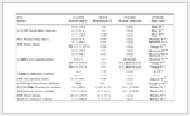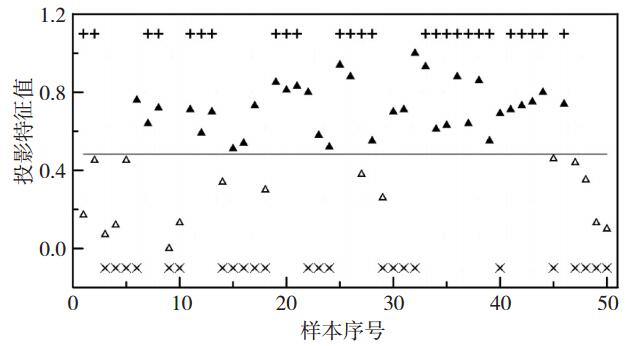﻿ 基于投影寻踪动态聚类模型的川东北雷暴预警的分析
 期刊检索： 题目 作者 关键词
 暴雨灾害2018, Vol. 37Issue (6): 582-586.  DOI: 10.3969/j.issn.1004-9045.2018.06.012DOI

10.3969/j.issn.1004-9045.2018.06.012

文章历史

1. 成都信息程大学大气科学学院 高原大气与环境四川省重点实验室, 成都 610225;
2. 巴中市气象局, 巴中 636000

Analysis of thunderstorm warning in northeastern Sichuan based on projection pursuit dynamic cluster model
, NI Changjian1, ZHU Yulei1, ZHENG Yunhua2
1. College of Atmospheric Sciences, Chengdu University of Information Technology, Plateau Atmosphere and Environment Key Laboratory of Sichuan Province, Chengdu 610225;
2. Bazhong Meteorological Bureau, Bazhong 636000
Abstract: Based on data of lightning locations and sounding observation data in Dazhou from October 2015 to September 2016, taking temperature difference between 850 hPa and 500 hPa, precipitable water, K Index, convective available potential energy, convective inhibition energy and lifting index as thunderstorm warning factors, the projection pursuit dynamic cluster method is used to construct the warning model for thunderstorms in the period. The results are as follows. (1) The critical success index for quantitative evaluation of model prediction results was 72%, and the case mentioned above was identified by this method. (2) Compared to conventional warning methods, this model has advantages of high recognition rate, simple calculation and objectivity. The model provides a new objective method for thunderstorm warning.
Key words: thunderstorm warning    forecast factor    PPDC    model

1 资料与方法 1.1 资料说明

1.2 投影寻踪动态聚类模型简介

 ${x_{ij}} = \frac{{x_{ij}^0-x_{j\min }^0}}{{x_{j\max }^0-x_{j\min }^0}}$ (1)

 ${x_{ij}} = \frac{{x_{j\max }^0-x_{ij}^0}}{{x_{j\max }^0-x_{j\min }^0}}$ (2)

 ${z_i} = \sum\limits_{j = 1}^m {{a_j}{x_{ij}}} \left( {i = 1, 2 \cdots, n} \right)$ (3)

（1）随机选取p个点，并作为p个聚核，记为L0 =(A10A20，...，AP0)；

（2）以L0把Ω中的点分为p类，记做Θ0 = (Θ10，Θ10，...，Θp0)。其中Θi0 ={z∈Ω|d(Ai0 - z) ≤ d(Aj0 - z)，∀j = 1，2，...，pji}，d(Ai0 - z)为点Ai0和集合Ω中任一点的绝对值距离。

（3）由Θ出发，对新的聚核L1L1 =(A11A21，...，AP1)加以计算。其中，$A_i^1 = \frac{1}{{{n_i}}}\sum\limits_{{z_i} \in \Theta _i^0}^p {{z_i}}$，类Θi0中有ni个点。

（4）重复以上步骤，由此得到一个分类结果序列${ \vee ^k} = \left( {{L^k}, {\Theta ^k}} \right), k = 1, 2, \cdots $$D\left( {A_i^k, \Theta _i^k} \right) = \sum\limits_{{z_i} \in \Theta _i^k} {\left| {{z_i}-A_i^k} \right|}$${U_k} = \sum\limits_{i = 1}^p {D\left( {A_i^k, \Theta _i^k} \right)}$，若$\frac{{\left| {{U_{k + 1}}-{U_k}} \right|}}{{{U_{k + 1}}}} \le \varepsilon$，则终止算法。其中，允许误差范围的充分小量用ε表示。这种算法理论证明是收敛的。

ss(a)和dd(a)的定义为依据，投影寻踪动态聚类模型的投影指标QQ(a)为

 $QQ\left( a \right) = ss\left( a \right)-dd\left( a \right)$ (4)

 $\begin{array}{l} {\rm{Min}}\;\;\;\;QQ\left( {\vec a} \right)\\ s.t.\;\;\;\;\sum\limits_{j = 1}^m {{a_j} = 1} \end{array}$ (5)

2 指标选取

3 应用实例表 1 川东北雷暴样本的预报因子及对应的特征值 Table 1 Predictors and corresponding projection values of thunderstorm samples in northeast Sichuan图 1 雷暴样本投影特征值的散点图(+表示实测有雷暴发生，×表示实测无雷暴发生，▲表示模型结果有雷暴发生，△表示模型结果无雷暴发生) Fig. 1 Scatter points of projection value of thunderstorm samples (+ Indicates that a thunderstorm occurred, × indicates that no thunderstorm occurred, ▲ indicates that a thunderstorm occurs in the model result, and Δ indicates that a thunderstorm does not occur in the model result)

4 结论与讨论

(1) 模型预报结果定量评估，命中率POD为92.31%，虚警率FAR为23.40%，临界成功指数CSI为72.00%，表明该模型对川东北雷暴潜势预报是有效、合理的。

(2) 与常规预警方法相比，该模型具有识别率高，计算简便，客观性强等特点，为雷暴预警提供一种新的客观方法。但由于雷暴预报的复杂性，上述模型有待进一步完善，如增加物理量预报因子，提高CSI评分。另外，模型虽然给出了有、无雷暴的特征值结果，然而在预报和实测中还存在雷暴强度和等级划分，鉴于此，可考虑进行雷暴强度的分级预报模型研究。

  朱乾根, 林锦瑞, 寿绍文, 等. 天气学原理和方法[M]. 北京: 气象出版社, 2000.  陈洪滨, 朱彦良. 雷暴探测研究的进展[J]. 大气科学, 2012, 36(2): 411-422.  陈雷, 戴建华, 汪雅. 近10 a长三角地区雷暴天气统计分析[J]. 暴雨灾害, 2015, 34(1): 80-87. DOI:10.3969/j.issn.1004-9045.2015.01.011  俞小鼎, 周小刚, 王秀明. 雷暴与强对流临近天气预报技术进展[J]. 气象学报, 2012, 70(3): 311-337. DOI:10.3969/j.issn.1004-4965.2012.03.003  郝莹, 姚叶青, 陈焱, 等. 基于对流参数的雷暴潜势预报研究[J]. 气象, 2007, 33(1): 51-56.  胡晓, 蒋飞燕, 徐璐, 等. 基于不稳定参数统计的雷暴潜势预报方法研究[J]. 浙江气象, 2016, 37(3): 11-17. DOI:10.3969/j.issn.1004-5953.2016.03.003  王秀明, 俞小鼎, 周小刚. 雷暴潜势预报中几个基本问题的讨论[J]. 气象, 2014, 40(4): 389-399. DOI:10.3969/j.issn.1000-6362.2014.04.005  田琨, 郭凤霞, 曾庆峰, 等. 南京地区雷暴活动强度潜势预报[J]. 气象科技, 2013, 41(1): 177-183. DOI:10.3969/j.issn.1671-6345.2013.01.032  Friedman J H, Tukey J W. A Projection Pursuit Algorithm for Exploratory Data Analysis[J]. IEEE Transactions on Computers, 2006, 23(9): 881-890.  David M.Glover. Exploration of multivariate atmospheric particulate compositional data by projection pursuit[J]. Atmospheric Environment, 1994, 28(8): 1411-1424. DOI:10.1016/1352-2310(94)90204-6  倪长健, 崔鹏. 投影寻踪动态聚类模型[J]. 系统工程学报, 2007, 22(6): 634-638. DOI:10.3969/j.issn.1000-5781.2007.06.012  王顺久. 水资源评价的投影寻踪动态聚类模型[J]. 四川大学学报:工程科学版, 2008, 40(5): 22-26.  张鹏洲, 倪长健. 基于投影寻踪动态聚类模型的边坡稳定性评价[J]. 四川环境, 2010, 29(1): 126-129. DOI:10.3969/j.issn.1001-3644.2010.01.028  Hagen M, Bartenschlager B, Finke U. Motion characteristics of thunderstorms in southern Germany[J]. Meteorological Applications, 1999, 6(3): 227-239. DOI:10.1017/S1350482799001164  张坚, 袁松, 姚叶青. 江淮地区雷暴的闪电定位系统与人工观测的一致性分析[J]. 暴雨灾害, 2015, 34(3): 286-292. DOI:10.3969/j.issn.1004-9045.2015.03.013  倪长健, 丁晶, 李祚泳. 免疫进化算法[J]. 西南交通大学学报, 2003, 38(1): 87-91. DOI:10.3969/j.issn.0258-2724.2003.01.020  陈勇, 匡方毅, 肖波. 基于Web GIS的长沙市雷暴天气短期预报模型的研究与应用[J]. 暴雨灾害, 2008, 27(3): 258-263. DOI:10.3969/j.issn.1004-9045.2008.03.011